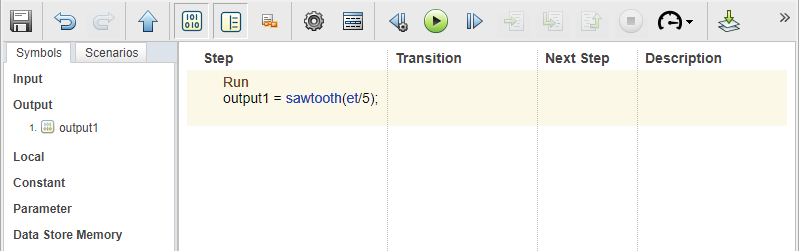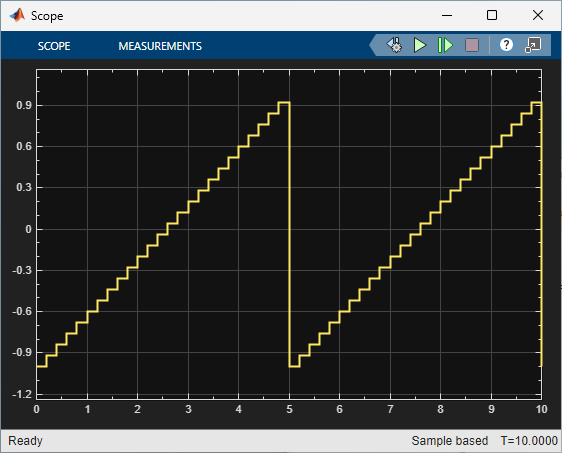# sawtooth

Sawtooth wave test signal

## Syntax

``y = sawtooth(x)``

## Description

example

````y = sawtooth(x)` creates a sawtooth wave from the input `x` based on the equation, $2\left(x-⌊x⌋-\frac{1}{2}\right),$where the L-brackets represent the floor of the contents. The output wave has a range from -1 to 1. Use the `et` operator as the input to generate the wave throughout simulation time in the test step. Divide `et` by a value to specify a period. Use the `sawtooth` operator in the test step of a Test Sequence or Test Assessment block.```

## Examples

expand all

In a Test Sequence block, create a sawtooth wave with a period of 5.The Test Sequence block outputs the sawtooth wave:## Input Arguments

expand all

Value during the test step, specified as a numeric scalar.

Data Types: `single` | `double` | `int8` | `int16` | `int32` | `int64` | `uint8` | `uint16` | `uint32` | `uint64`

## Version History

Introduced in R2015a